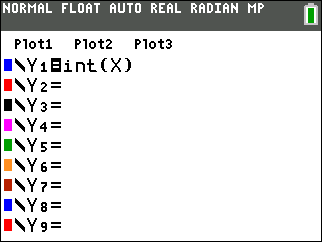Graphing the Greatest Integer Function

 The Greatest Integer Function is denoted by y = [x]. For all real numbers, x, the greatest integer function returns the largest integer less than or equal to x. In essence, it rounds down a real number to the nearest integer. For example:    = 1      [1.5] = 1      [3.7] = 3      [4.3] = 4 Beware!    [-2] = -2      [-1.6] = -2      [-2.1] = -3      [-5.5] = -6 Calculator GraphThe command "int" is found under MATH → NUM #5:int( or find in the Catalog. Actual Graph• If copying the graph from your calculator, be sure to indicate the open and closed circles on the ends of the line segments. Also, look carefully at the scale locations of the segments is you are transferring the graph onto a sheet of graph paper.Finding Your Way Around TABLE of  CONTENTS